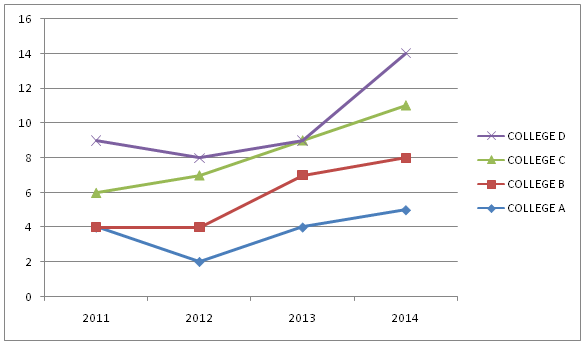# SBI Clerk Prelims 2021 Quantitative Aptitude Questions (Day-31)

Dear Aspirants, Our IBPS Guide team is providing new series of Quantitative Aptitude Questions for SBI Clerk Prelims 2020 so the aspirants can practice it on a daily basis. These questions are framed by our skilled experts after understanding your needs thoroughly. Aspirants can practice these new series questions daily to familiarize with the exact exam pattern and make your preparation effective.

Start Quiz

Approximation

Directions (01-04): Find out the approximate values comes in the place of (?) in the following questions.

1) 45.8% of 9502.20 – 1968.22 + 1682.43 =?

A.4294

B.6560

C.4080

D.4000

E.4120

2) 10.82 + 11.023 + 548.9 x 3.9 – ? = 1212

A.30

B.10

C.2168

D.2250

E.2440

3) 4564.12 ÷ 12 + 1453.22 – 782 = ? + 589.10

A.460

B.315

C.650

D.478

E.523

4) √728.82 +√ 3025.33 ÷ 5.22 + ? ÷ 8 = 975.550

A.7200

B.7250

C.7140

D.7500

E.7940

Data Interpretation

Directions (05-10): Study the following information carefully and answer the questions given below:

The following line graph represents the number of students in different Engineering colleges A, B, C and D in the year 2011 to 2014. The number of students is in lakh.5) What is the sum of the total number of students in the college A, B, C and D in the year 2014?

A.3445000

B.3550000

C.3700000

D.3800000

E.3654000

6) What is the difference between the sum of the no. of students in college C and D in the year 2013 and the sum of the no. of students in college A and B in the year 2012?

A.1420000

B.1450000

C.1200000

D.1242500

E.1440200

7) What will be the ratio of the total no. of students of college A and C in 2013 to the total no. of students of college B and D in the same year?

A.13: 16

B.26: 8

C.28: 7

D.27: 7

E.26: 9

8) Find the average of the total no. of student of all the college in the year 2013.

A.700000

B.882000

C.120000

D.725000

E.187600

9) What is the approximate percentage of the total no. of students in college B in 2012 out of the total no. of students in all the colleges in 2012?

A.16%

B.11%

C.19%

D.24%

E.22%

10) If the ratio of science to commerce students is 7: 9 in college A in 2014 and the ratio of science to commerce students is 5:4 in college D in 2013, then find the ratio of the number of science students of college A in 2014 to the number of science students of college D in 2013.

A.7: 18

B.6: 21

C.5: 16

D.8: 19

E.7: 16

45.8% of 9502.20 – 1968.22 + 1682.43 =?

46/100 x 9500 – 1968 + 1682 = ?

? = 4370 – 1968 + 1682 = 4084 = 4080 approx.

10.82 + 11.023 + 548.9 x 3.9 – ? = 1212

11+ 11+ 549 x 4 – 1212 = ?

121 + 1331 + 2196 – 1212 = 2436 = ?

? = 2440 approx

4564.12 ÷ 12 + 1453.22 – 782 = ? + 589.10

4564/12 + 1453 –782 – 589 = ?

380 + 1453 – 782 – 589 = ?

462 = ?

? = 460 approx.

√728.82 +√ 3025.33 ÷ 5.22 + ? ÷ 8 = 975.550

√729 + √3025 ÷ 5 +? ÷ 8 = 976

27 + 55/5 + ?/8 = 976

38 + ?/8 = 976

? = 7504 = 7500 approx.

Total students (A + B + C + D) in 2014 = (5 + 8 + 11 + 14) X 100000

= 3800000

No. of students (A + B) in 2012 = 400000 + 200000 = 600000

No. of students (C + D) in 2013 = 1800000

Difference = 1800000 – 600000 = 1200000

Required ratio = (4 + 9): (7 + 9) = 13: 16

Total students of (A + B + C + D) in 2013 = 2900000

Average = 2900000/4 = 725000

Approximate % = 400000/(200000 + 400000 + 700000 + 800000) x 100

= 19.04 = 19% approx.

Total students in college A in 2014 = 500000

Total students in college D in 2013 = 900000

Science students in college A in 2014 = 500000 x 7/16 = 218750

Science students in college D in 2013 = 900000 x 5/9 = 500000

Required ratio = 218750: 500000 = 7: 16

 Check Here to View SBI Clerk Prelims 2021 Quantitative Aptitude Questions Day – 30 Day – 29 Day – 28